Courses

# Introduction: The Force Method of Analysis - 3 Civil Engineering (CE) Notes | EduRev

## Civil Engineering (CE) : Introduction: The Force Method of Analysis - 3 Civil Engineering (CE) Notes | EduRev

The document Introduction: The Force Method of Analysis - 3 Civil Engineering (CE) Notes | EduRev is a part of the Civil Engineering (CE) Course Structural Analysis.
All you need of Civil Engineering (CE) at this link: Civil Engineering (CE)

Summary

The force method of analysis may be summarized as follows.

Step 1. Determine the degree of statical indeterminacy of the structure. Identify the redundants that would be treated as unknowns in the analysis. Now, release the redundants one by one so that a statically determinate structure is obtained. Releasing the redundant reactions means removing constraint corresponding to that redundant reaction. As in the above propped cantilever beam, either reactions RB or MA can be treated as unknown redundant. By choosing RB as the redundant, the propped cantilever beam can be converted into a cantilever beam (statically determinate) by releasing the roller support. Similarly by choosing moment as the redundant reaction, the indeterminate structure can be released into a determinate structure (i.e. a simply supported beam) by turning the fixed support into a hinged one. If the redundant force is an internal one, then releasing the structure amounts to introducing discontinuity in the corresponding member. The compatibility conditions for the redundant internal forces are the continuity conditions. That would be discussed further in subsequent lessons.

Step 2. In this step, calculate deflection corresponding to redundant action, separately due to applied loading and redundant forces from force displacement relations. Deflection due to redundant force cannot be evaluated without knowing the magnitude of the redundant force. Hence, apply a unit load in the direction of redundant force and determine the corresponding deflection. Since the method of superposition is valid, the deflections due to redundant force can be obtained by simply multiplying the unknown redundant with the deflection obtained from applying unit value of force.

Step 3. Now, calculate the total deflection due to applied loading and the redundant force by applying the principle of superposition. This computed total deflection along the redundant action must be compatible with the actual boundary conditions of the original structure. For example, if in the original structure, the deflection corresponding to the redundant reaction is zero then the total deflection must be equal to zero. If there is more than one redundant force then one could construct a set of equations with redundant forces as unknowns and flexibility coefficients as coefficients of the equations. The total number of equations equals the number of unknown redundants.

Step 4. In the last step, evaluate all other reactions and internal forces from the equilibrium equations.

The method of superposition or the force method as discussed above is applied to any type of structures, i.e. beams, truss and frames or combination of these structures. It is applicable for all general type of loadings.

The deflection of statically determinate structure can be obtained by unit-load method or by moment-area theorem or by any method known to the reader. However, the deflections of few prismatic beams with different boundary conditions and subjected to simple loadings are given in Fig. 7.4. These values will be of help in solving the problems of the present and subsequent lessons. However the students are strongly advised to practice deriving them instead of simply memorizing them.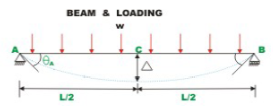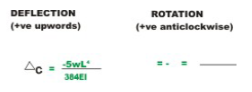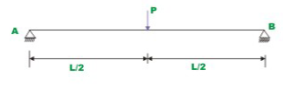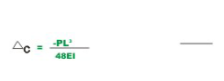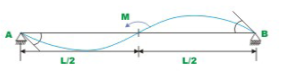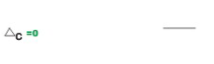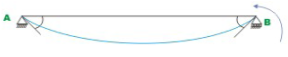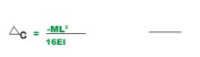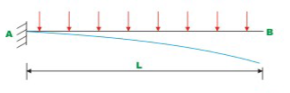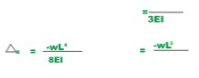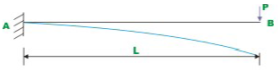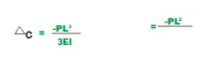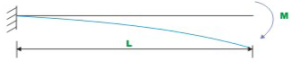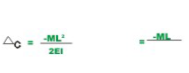Example 7.1

A continuous beam ABC is carrying a uniformly distributed load of 1 kN/m in addition to a concentrated load of 10 kN as shown in Fig.7.5a, Draw bending moment and shear force diagram. Assume EI to be constant for all members.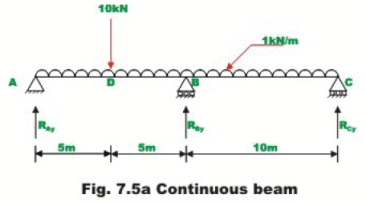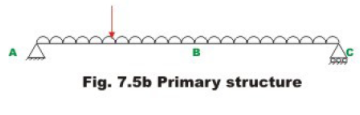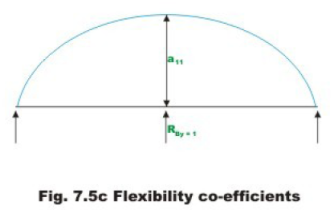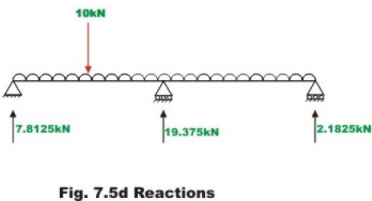It is observed that the continuous beam is statically indeterminate to first degree. Choose the reaction at B, RBy as the redundant. The primary structure is a simply supported beam as shown in Fig.7.5b.

Now, compute the deflection at B, in the released structure due to uniformly distributed load and concentrated load. This is accomplished by unit load method. Thus,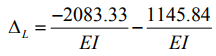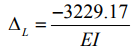(1)

In the next step, apply a unit load at B in the direction of RBy (upwards) and calculate the deflection at B of the following structure. Thus (see Fig. 7.5c),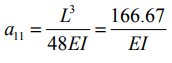(2)

Now, deflection at B in the primary structure due to redundant RB is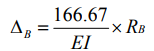(3)

In the actual structure, the deflection at B is zero. Hence, the compatibility equation may be written as

ΔL + ΔB = 0                                   (4)

Substituting for ΔL and ΔB in equation (4),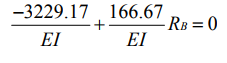(5)

Thus,

RB = 19.375 kN

The other two reactions are calculated by static equilibrium equations (vide Fig. 7.5d)

RA = 7.8125 kN
RB = 2.8125 kN

The shear force and bending moment diagrams are shown in Fig. 7.5e and Fig. 7.5f respectively.

Example 7.2

A propped cantilever beam AB is subjected to a concentrated load of 60 kN at 3m from end A as shown in Fig. 7.6a. Draw the bending moment and shear force diagrams by the force method. Assume that the flexural rigidity of the beam, EI to be constant throughout.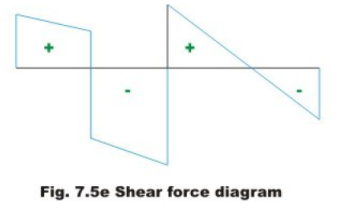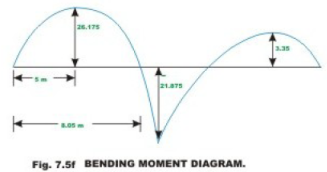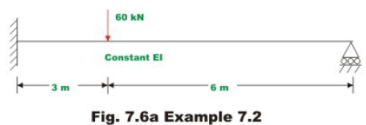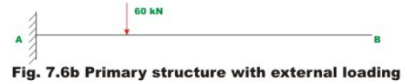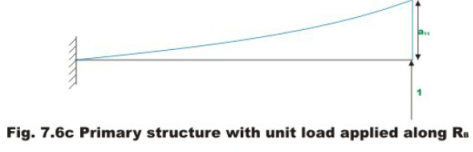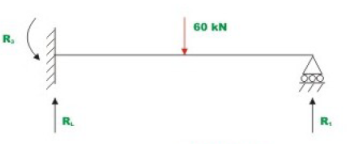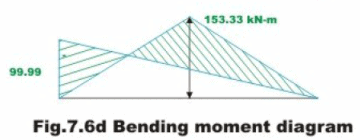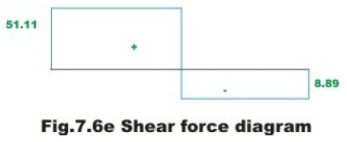The given problem is statically indeterminate to first degree. Choose the reaction at B, R1 as the redundant. After releasing the redundant, the determinate structure, a cantilever beam in this case is obtained. The cantilever beam with the applied loading is chosen in Fig 7.6b.

The deflection of the released structure is,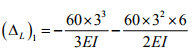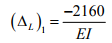(1)

The deflection at point B due to unit load applied in the direction of redundant R1 is (vide Fig 7.6c)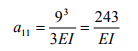(2)

Now the deflection at B due to redundant is R1 is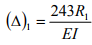(3)

From the original structure it is seen that the deflection at B is zero. Hence, the compatibility condition for the problem may be written as,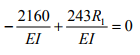(4)

Solving equation (4), the redundant R1 is obtained.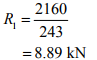(5)

The vertical reaction and fixed end moment at A can be determined from equations of statics. Thus,

R2 = 51.11 kN

R3 = 99.99 kN.m                 (6)

Shear force and bending moment diagrams are shown in Fig. 7.6d and Fig. 7.6e respectively.

Summary

In this lesson flexibility matrix method or the method of consistent deformation or the force method of analysing statically indeterminate structures has been introduced with the help of simple problems. The advantages and limitations of flexibility matrix method have been discussed. Only simple indeterminate beam problem has been solved to illustrate the procedure. The principle of superposition has been used to solve statically indeterminate problems.

Offer running on EduRev: Apply code STAYHOME200 to get INR 200 off on our premium plan EduRev Infinity!

## Structural Analysis

30 videos|122 docs|28 tests

,

,

,

,

,

,

,

,

,

,

,

,

,

,

,

,

,

,

,

,

,

;